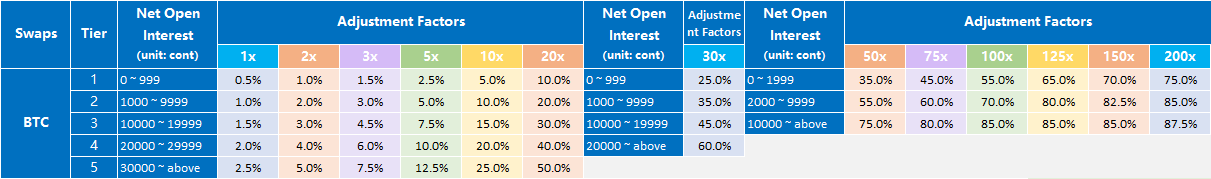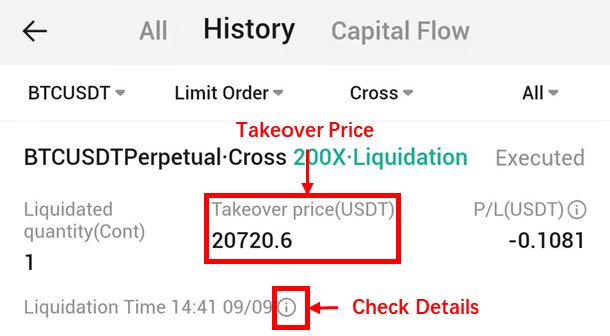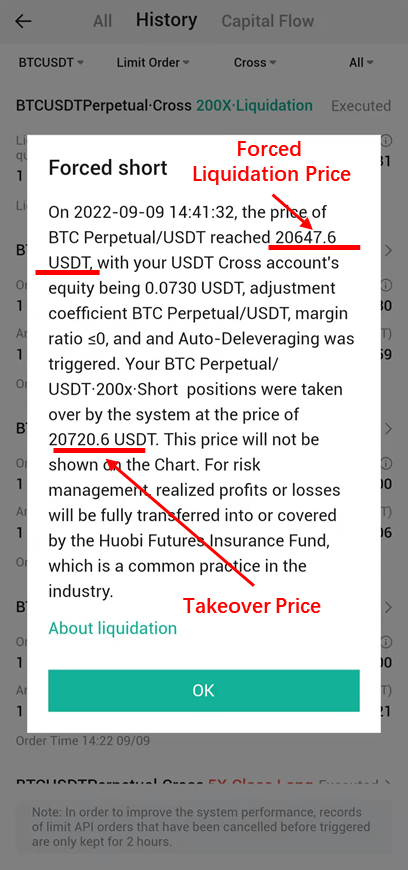Forced Liquidation of Huobi

# 1. Overview of forced liquidation system

The forced liquidation system is an important crisis management system for Huobi. When your trading margin is insufficient and is not replenished within the specified time or your position exceeds the specified limit, in order to prevent the expanding crisis, the open positions you hold will be forced to liquidate, which is called forced liquidation system.

# 2. Liquidation introduction

## （1）Margin Ratio

Margin ratio is an indicator used to estimate the users’ assets risk. When margin ratio is less than or equal to 0%, forced liquidation will be triggered.

Margin Ratio = ( Account Equity / Used Margin ) * 100% – Adjustment Factor

Note: Used Margin = Position Margin + Frozen Margin

Huobi Futures implement tiered liquidation. That is, the system will try to reduce the gear corresponding to the Adjustment Factors, so as to avoid the position being liquidated all at once.

If the liquidation is triggered when the gear corresponding to the Adjustment Factors is 1st level: The system will cancel all current orders for this symbol contract;

The long and short positions of the contract of the same symbol will be self-traded;

If the margin ratio of the user's position is still less than 0 at this time, it will be liquidated at once time.

If the liquidation is triggered when the gear corresponding to the Adjustment Factors is 1st level:

The system will cancel all current orders for this symbol contract;

The long and short positions of the contract of the same symbol will be self-traded;

If the margin ratio is still less than 0, the system will reduce the adjustment factor for the purpose of forcibly reducing the position to the upper limit of the net position of a certain position, and the margin rate is more than 0;

If the margin reduction is forced to the 1st level and the margin ratio is still not more than 0, then all remaining positions will be liquidated at once time.

There is no charge for partial liquidation. Users cannot perform operations related to this type of contract when the forced liquidation is triggered.

## （2）Estimated Liquidation Price

The estimated liquidation price refers to the market price when the guaranteed asset ratio = 0% (this price is only for investors' investment reference). The actual forced liquidation price is based on the latest transaction price when the guaranteed asset ratio is ≤0%.

The adjustment factor is designed to prevent users from losing margin calls on the system. Huobi Futures uses a layered adjustment factor mechanism, which supports up to five levels most depending on the currency. There will be in a higher adjustment factor coefficient for a higher position.

According to the table below, if a user has 1000 lots of BTC contracts in open interest, Corresponds to the 2nd level. The adjustment factor for 10 leverage multiple, 20 leverage multiple and 5 leverage multiple is 10%, 20% and 5% correspondingly.[The above data and indicator contents may be adjusted in real-time according to market conditions, and the adjustments will be made without further notice.]

## （4）Take-over after the liquidation

The liquidation price is the market price for reference when the margin ratio is equal to or less than 0%. When the margin ratio reaches or below 0%, liquidation will be triggered and the trading system will take over the positions with a limited order price. Since the taken-over positions won’t go through the match system, the price of the taken-over positions will not display on the market (K-line chart). Please note that the liquidation order price is different from the liquidation price. For liquidation order details, you can go to Huobi APP and click [ ? ] or go to the web page and click “Details” in “Order History”.Details of liquidated orders:## （5）Example

Suppose Bob has 20 BTC in account equity. He opened a long 15000-lot BTC swap contract at the price of 8000USD/BTC(100USD/lot). Assuming there was 10X in leverage, and 15% in adjustment factor, the corresponding gear is the 3rd level. Do not have corresponding transaction fees as well, what will happen to Bob’s positions when the last BTC swap contract reaches 7337.3 USD?

Let’s have a look at the calculation for different elements.

1. Unrealized Profit and Losses

According to the order direction-open long, the formula is Unrealized P/L for long position= (1 / Position Price – 1 / last order price) * order quantity * contract face value

Therefore, the calculation for Bob’s Unrealized P/L is: (1 / 8000 – 1 / 7,337.3) * 15000 * 100 = –16.9348BTC

In this case, when the price of BTC swap contract reaches 7330.12 USD, Bob’s unrealized profit and losses is –16.9348BTC

1. Bob’s account equity

Formula: Equity = Deposit + realized profits / losses + unrealized profits / losses

Bob’s equity is 20 + 0 + (–16.9348 BTC) = 3.0652BTC

Therefore, when the price of BTC swap contract reaches 7337.3 USD, Bob’s account equity is 3.0652BTC.

1. Position Margin

Formula: Position Margin = (Contract Value * Position Quantity) / Last Order Price / Leverage

Bob’s position Margin is (100 * 15000) / 7,337.3 / 10 = 20.4434BTC

Therefore, when the price of BTC swap contract reaches 7330.12, Bob’s current position margin is 20.4434BTC

1. Is liquidation triggered？

Formula: Margin Ratio = (Account Equity / Used Margin) * 100% – Adjustment Factor

Bob’s margin ratio is (3.0652 / 20.4434) * 100% – 15% = 0%

In this case, the EMA price according to the EMA formula is 7337.3

As explained above, when the margin ratios calculated according to both the last price and the EMA go to or below 0, Bob’s order will be liquidated

Therefore, Bob’s order is liquidated when the price of BTC swap contract reaches 7337.3 USD

1. What will happen after liquidation？

After the liquidation triggered, the system detects that Bob's net position is 15,000 and the scale corresponding to the adjustment factor is the 3rd gear. Then the system will try to recalculate Xiaoming's margin rate using the maximum value of the 2nd gear of 9999 sheets as the remaining position amount and the corresponding adjustment factor of 12.5%;

Position Margin = (100 * 9999) / 7,337.3 / 10 = 13.6276BTC

Realized profits / losses of positions that be takeover：( 1 / 8000 – 1 / 7228.9 ) * （ 15000 – 9999 ）* 100 = –6.6681BTC

Unrealized profits / losses of positions that not be takeover：( 1 / 8000 – 1 / 7,337.3 ) * 9999 * 100 = –11.2887BTC

Account equity = 20 + （ – 6.6681 ） + ( – 11.2887 ) = 2.0432 BTC

Therefore, if Bob only holds 9999 BTC contracts, the margin ratio of his position = ( 2.0432 / 13.6276 ) * 100% – 12.5% ＞ 0%

At this time, the system will take over more than 15000 – 9999 = 5001 contracts beyond the second level by the system at the takeover price, which means that the releases partial liquidation of Bob's partial liquidation.

1. How about the Liquidation Orders Price（Takeover Price）？

As mentioned above, when liquidation is triggered, the trading system will take over the order with a limit order price when the account equity goes to 0, Let us calculate the liquidation order price（takeover price）. At first, we set the liquidated price to x, and then set the specific value to see:

Because (1 / 8000 – 1 / x) * 15000 * 100 = –20 BTC

So x = 7228.9

That the price is 7228.9 USD when Xiaoming's account equity is 0. At the same time, this price is also the price at which the system takes over Bob's 5001 positions. The liquidation order price will not be displayed on the K-line;

After the tiered force liquidation, Bob's remaining position is 9999 BTC swap contracts, and relevant operation authority will be restored.

（The above is a reasonable example use, the specific settings or related migration are subject to the platform announcement）

# 3. Expense Sharing after Liquidation

## （1）Insurance fund

An insurance fund is set up to cover societal losses (i.e. losses attributed to unfilled liquidation orders) or to settle incidents in contract trading.

For each digital asset contract, there is a corresponding insurance fund, and the same digital asset contracts of different periods share one insurance fund.

The sources of insurance funds are mainly from Huobi Futures and the premiums after forced liquidations. Once forced liquidation is triggered, the system would take over users’ positions and close them by placing orders on the order book. The premiums generated by liquidated positions will be injected into the corresponding insurance fund. In terms of initial trade or special situations, the system will manually transfer the premiums (generated by liquidated positions) into the exchange account, supplementing the insurance fund.

A small percentage of fees may be charged when the system takes over users’ positions and implements the liquidation.

Use of insurance fund: During the settlement period of each cycle, if there were margin call losses attributed to unfilled liquidation orders, the system would first use the insurance fund to meet the losses. The clawback will only occur if the insurance fund is not enough to cover the system's total margin call losses.

## （2）Claw back

When the market fluctuates severely, the user is subjected to liquidation, and the transaction cannot be concluded at the liquidation price, resulting in loss greater than the margin, the platform will adopt the “clawback” system. The clawback will only occur if the insurance fund does not have enough funds to cover the system's total margin call losses.

## （3）Full account clawback system

The system's total margin call losses

If system's losses + insurance fund >= 0, then claw back rate = 0

If system's losses + insurance fund < 0, then claw back rate = (system losses + insurance fund) / net profit across all contracts

Clawback coefficient = System's losses / Profit across all contracts

For example:

At the time of settlement, the BTC perpetual swaps had a total loss of -120 BTC.

First fill it with risk reserves, if there is still a loss of -20BTC after filling. It needs to be allocated from the BTC contract profit account.

Assuming all the profits of the profitable account are 400000BTC, the allocation factor is 20/400000 = 1/20000

The perpetual swaps of an account made a total profit of 2BTC in this period, the amount to be allocated for this account is 2 * (1/20000) = 0.0001BTC.